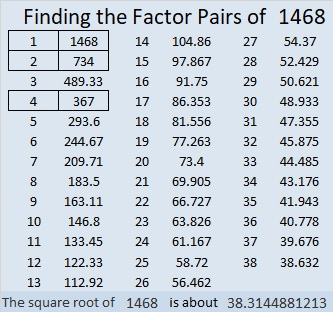# 1468 and Level 1

Contents

### Today’s Puzzle

Can you find the factors from 1 to 12 that will work with the given clues to turn this puzzle into a multiplication table?### Factors of 1468

• 1468 is a composite number.
• Prime factorization: 1468 = 2 × 2 × 367, which can be written 1468 = 2² × 367
• 1468 has at least one exponent greater than 1 in its prime factorization so √1468 can be simplified. Taking the factor pair from the factor pair table below with the largest square number factor, we get √1468 = (√4)(√367) = 2√367
• The exponents in the prime factorization are 2 and 1. Adding one to each exponent and multiplying we get (2 + 1)(1 + 1) = 3 × 2 = 6. Therefore 1468 has exactly 6 factors.
• The factors of 1468 are outlined with their factor pair partners in the graphic below.### Another Interesting Fact about the number 1468:

OEIS.org informs us that the smallest 20-digit 6th power is 1468⁶.

This site uses Akismet to reduce spam. Learn how your comment data is processed.